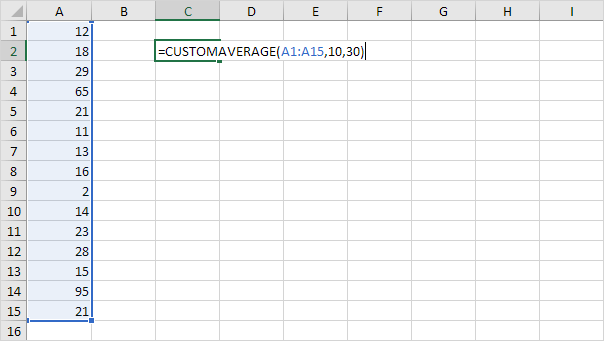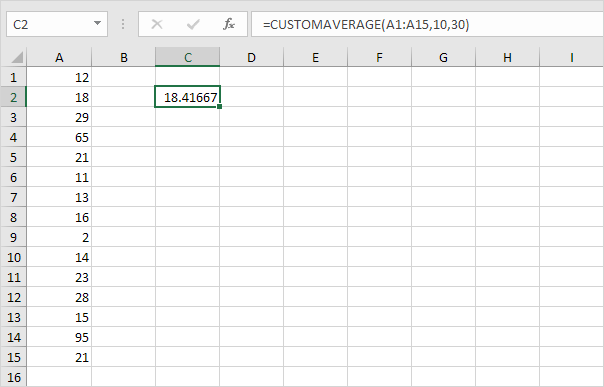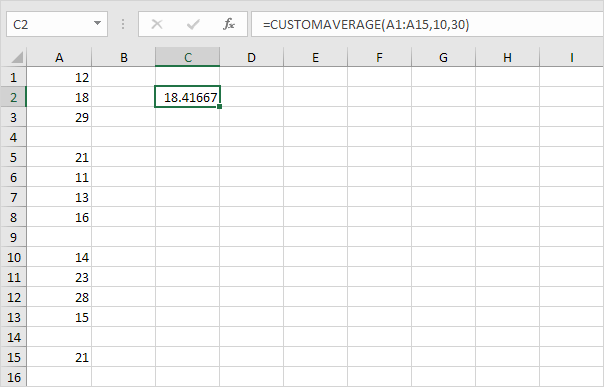# Custom Average Function

Below we will look at a program in Excel VBA which creates a User Defined Function that calculates the average of a randomly selected range excluding one or more values that are outliers and shouldn't be averaged.

Situation:User defined functions need to be placed into a module.

1. Open the Visual Basic Editor and click Insert, Module.

2. Add the following code line:

Function CUSTOMAVERAGE(rng As Range, lower As Integer, upper As Integer)

The name of our Function is CUSTOMAVERAGE. The part between the brackets means we give Excel VBA a range and two Integer variables as input. We name our range rng, one Integer variable we call lower, and one Integer variable we call upper, but you can use any names.

3. Next, we declare a Range object and two variables of type Integer. We call the Range object cell. One Integer variable we call total and one Integer variable we call count.

Dim cell As Range, total As Integer, count As Integer

4. We want to check each cell in a randomly selected range (this range can be of any size). In Excel VBA, you can use the For Each Next loop for this. Add the following code lines:

For Each cell In rng

Next cell

Note: rng and cell are randomly chosen here, you can use any names. Remember to refer to these names in the rest of your code.

5. Next, we check for each value in this range if it falls between the two values (lower and upper). If true, we increment total by the value of the cell and we increment count by 1. Add the following code lines to the loop.

If cell.Value >= lower And cell.Value <= upper Then
total = total + cell.Value
count = count + 1
End If

6. To return the result of this function (the desired average), add the following code line outside the loop.

CUSTOMAVERAGE = total / count

7. Don't forget to end the function. Add the line:

End Function

8. Now you can use this function just like any other Excel function to calculate the average of numbers that fall between two values.

Result:As a check, you can delete all values that are lower than 10 and higher than 30 and use the standard Average function in Excel to see if Excel calculates the same average as our custom average function.Our custom average function works! Note: this function is only available in this workbook.

Go to Next Chapter: Application Object